Quick Contact# Simple harmonic Motion

### Kinematics of simple harmonic motion

4.1.1 Describe examples of oscillations

• A pendulum
• A mass attached to a spring attached to a wall that oscillates back and forth.

## 4.1.2 Define the terms displacement, amplitude, frequency, period and phase difference.

Displacement - The instantaneous distance of the moving object from its mean position

Amplitude - The maximum displacement achievable from the mean position

Frequency - The number of oscillations completed per unit time

F = 1/t

Period - the time taken for a complete oscillation

T = 1/f

Phase difference - the measure of how "in step" different particles are. If they are moving together they are said to be in phase. If not they are said to be out of phase.

## 4.1.3 Define simple harmonic motion (SHM) and state the defining equation as a = −ω²x.

Simple harmonic motion is defined as the motion that takes place when the acceleration, a , is always directed towards and is proportional to its displacement from a fixed point.

The acceleration is caused by a restoring force that always pointing to the mean position and is proportional to the displacement from the mean position.

a = −ω²x

The negative signs signifies that the acceleration is always is always pointing back towards the mean position

## 4.1.5 Apply the equations x = sin⁡(ωt) , v = Aωcos(ωt), a = -Aω^2  sin⁡(ωt), v =±ω√((A^2-x^2 ) ) as solutions to the defining equation for SHM.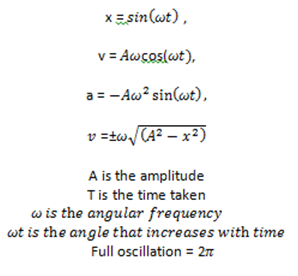## Elastic potential energy EEP = 1/2 kx2## 4.1.6 Solve problems, both graphically and by calculation, for acceleration, velocity and displacement during SHM.

### Energy changes during simple harmonic motion (SHM)

4.2.1 Describe the interchange between kinetic energy and potential energy during SHM.

In a SHM motion the total energy is interchanged between kinetic energy and potential energy. If no resistive forced acts on the motion the total energy is constant and is said to be undamped.

Potential energy increases as we move away from the equilibrium position and kinetic energy decreases. As we come closer to the equilibrium position its vice versa. EP can be expressed as a sine curve, EK as a cosine curve.

## 4.2.2 Apply the expressions E  = 1⁄2 mω^2 (x_0^2-x^2 ) for the kinetic energy of a particle undergoing SHM, E = 1⁄2 mω^2 x_0^2 for the total energy and E =1⁄2 mω^2 x^2  for the potential energy.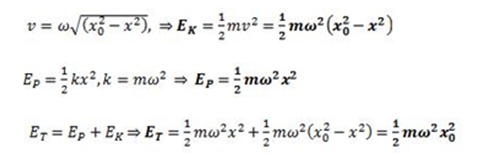## 4.2.3 Solve problems, both graphically and by calculation, involving energy changes during SHM.

### Forced oscillations and resonance

4.3.1 State what is meant by damping.

Damping is a dissipating force that is always in the opposite direction to the direction of motion of the oscillating particle. As work is being done against the dissipating force energy is lost. Since energy is proportional to the amplitude, the amplitude decreases exponentially with time.

## 4.3.2 Describe examples of damped oscillations.

Under damped -where there is  a small dissipating force and a fraction of the total energy is removed after every oscillation and hence the amplitude decreases.

Critical damped - this is when there is an intermediate dissipating force and the system reaches equilibrium position as fast as possible without oscillating.

Over damped - there is a large dissipating force and the system takes longer to reach equilibrium position than critical damping. There are no oscillations in over damping.

## 4.3.3 State what is meant by natural frequency of vibration and forced oscillations.

Natural frequency - the frequency at which the system vibrates when in motion

Forced oscillation- when an external force is applied to the original frequency causing a change in the frequency of the oscillation.

## 4.3.4 Describe graphically the variation with forced frequency of the amplitude of vibration of an object close to its natural frequency of vibration.

The amplitude of the forced oscillation depends on  comparative values of the natural frequency and the driving frequency. In addition it also depends on the amount of damping present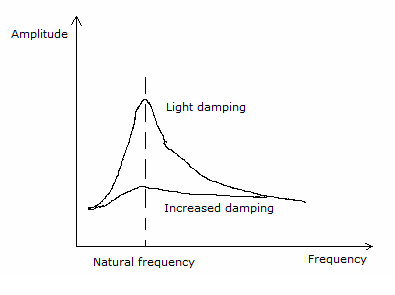Figure 4.3.1 - Variation with forced frequency of the amplitude of vibration of an object

## 4.3.5 State what is meant by resonance.

Resonance is when the natural frequency of a system is equal to the frequency of an external force. This results in oscillating and an increase in amplitude.

## 4.3.6 Describe examples of resonance where the effect is useful and where it should be avoided.

Quartz oscillators, microwave

Generators and vibrations in machinery.

### Wave Characteristics

4.4.1 Describe a wave pulse and a continuous progressive (travelling) wave.

A wave is a disturbance that propagates through some material medium or space.

Waves have the following characteristics:

• They all transfer energy
• They do this without a net motion
• They all involve oscillations that are SHM

A wave pulse involves just one oscillation

A continuous wave involves a succession of individual oscillations.

## 4.4.2 State that progressive (travelling) waves transfer energy.

Waves transfer energy without the net motion of the medium through which the wave travels.

## 4.4.3 Describe and give examples of transverse and of longitudinal waves.

Transverse waves are waves in which the oscillations of the medium are at right angles to the direction of the energy transfer, e.g. light waves.

Longitudinal waves are waves in which the oscillations are parallel to the direction of energy transfer, e.g. sound waves.

## 4.4.4 Describe waves in two dimensions, including the concepts of wavefronts and of rays.

Take for example a water wave. The wavefronts are the parts of the wave that are moving together. Rays represent the direction of the energy transfer.

## 4.4.5 Describe the terms crest, trough, compression and rarefaction.

Crest - The top, maximum height of the transverse wave

Trough - The bottom, the lowest point, of the transverse wave.

Compression - A point on the longitudinal wave that are "bunched together" (high pressure)

Rarefraction - A point on the longitudinal wave that are "far apart" (low pressure)

## 4.4.6 Define the terms displacement, amplitude, frequency, period, wavelength, wave speed and intensity.

Displacement: The amount by which a particle is moved from equilibrium position.

Amplitude: The maximum displacement of a particle from its equilibrium position.

Period: The time that it takes a particle to make one complete oscillation, or the time that it takes for the wave to travel a complete wavelength.

Wavelength: The shortest distance along a wave between two points that are "in phase" with one another. e.g the distance from one crest to another crest on a water wave.

Wave speed: The speed at which the wave fronts pass a stationary object.

Intensity: The intensity of the wave is the power per unit area that is received by a stationary observer.  intensity ∝ amplitude2.

## 4.4.8 Derive and apply the relationship between wave speed, wavelength and frequency.## 4.4.9 State that all electromagnetic waves travel with the same speed in free space, and recall the orders of magnitude of the wavelengths of the principal radiations in the electromagnetic spectrum.Figure 4.4.1 - The electromagnetic spectrum

## 4.5.1 Describe the reflection and transmission of waves at a boundary between two media.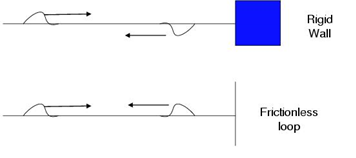Figure 4.5.1 - Transmission of waves

When fixed, the wave is reflected and inverted. When free, the wave is reflected.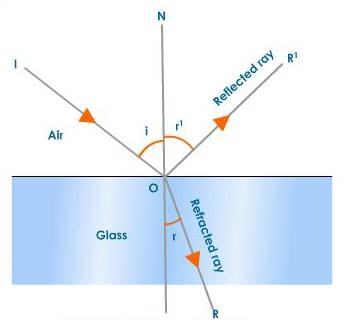Figure 4.5.2 - Reflection and refraction of waves

When the wave meets the boundary between two different mediums it is partially reflected and partially refracted.

## 4.5.2 State and apply Snell’s law.

The refraction indices, n, represent the factor by which light is slowed down within a refractive medium compared to its velocity in vacuum

## 4.5.3 Explain and discuss qualitatively the diffraction of waves at apertures and obstacles.

When waves pass through an aperture they tend to spread. This is similarly the case with obstacles. This wave property is known as diffraction. For there to be diffraction the wavelength needs to be of the same order of magnitude as the aperture width. This becomes more important as the wavelength of the wave is large as compared to the aperture width.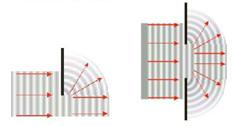Figure 4.5.3 - Diffraction of waves

## 4.5.4 Describe examples of diffraction.

Diffraction is the reason to why we can still hear sounds of objects without seeing them. You can hear the TV from upstairs in your room if it loud.

## 4.5.5 State the principle of superposition and explain what is meant by constructive interference and by destructive interference.

The principal of superposition states that when two waves meet the resultant displacement is the sum of the individual displacements.

Constructive interference occurs when two pulses displaced in the same direction cause an overlap. The resultant displacement is the sum of both displacements.

Destructive interference occurs when two pulses displaced in opposite directions  cause an overlap. The resultant displacement is the difference of both displacements.

## 4.5.6 State and apply the conditions for constructive and for destructive interference in terms of path difference and phase difference.

Constructive interference is when two waves are In phase. By that we mean the path difference between the two waves is zero

Destructive interference is when the two waves are out of phase

Content